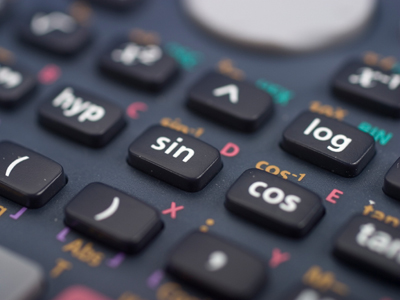See if you can get full marks in this math quiz.

# Algebra 1

This Math quiz is called 'Algebra 1' and it has been written by teachers to help you if you are studying the subject at high school. Playing educational quizzes is a user-friendly way to learn if you are in the 9th or 10th grade - aged 14 to 16.

It costs only \$12.50 per month to play this quiz and over 3,500 others that help you with your school work. You can subscribe on the page at Join Us

This high school Math quiz will test your knowledge of the basic rules of Algebra.

Algebra is all about using symbols, usually letters, to represent a number that we don’t yet know. We call these letters VARIABLES, as their value can change, or vary, depending on the situation. A situation can be written down as an EXPRESSION – this can be thought of as a mathematical sentence. There are a few rules you need to know so that these expressions can be as neat and tidy as possible.

Imagine you had a number of squares and quarter-circles, and you can combine these to make different shapes. We can use the letter S to indicate a square, and Q for a quarter-circle.

Using 3 squares and 2 quarter-circles it is possible to make a number of different shapes, but each one will have the same area. We can write this as the expression 3S + 2Q.

Now imagine someone else comes along and gives you 2 squares and 4 quarter-circles. How many squares, and how many quarter-circles do you now have? It should be obvious that you have 5S + 6Q. You can’t add a square to a circle, and one of our rules of Algebra is that we can’t add terms that have different letters. The rules for multiplying and dividing are a bit different, so make sure you know the difference.

Having good Algebra skills opens up a whole world of opportunities, from Finance to Space Travel and everything in between!

Question 1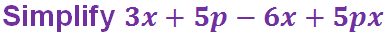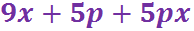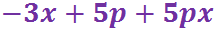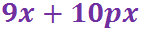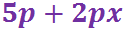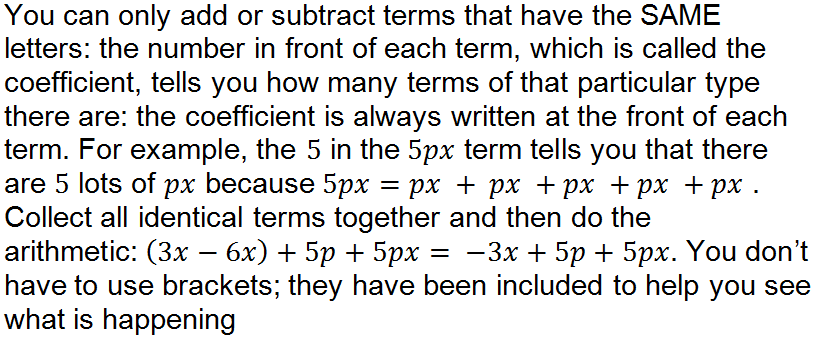Question 2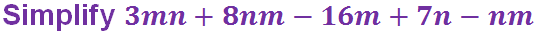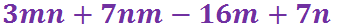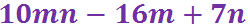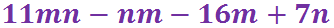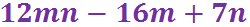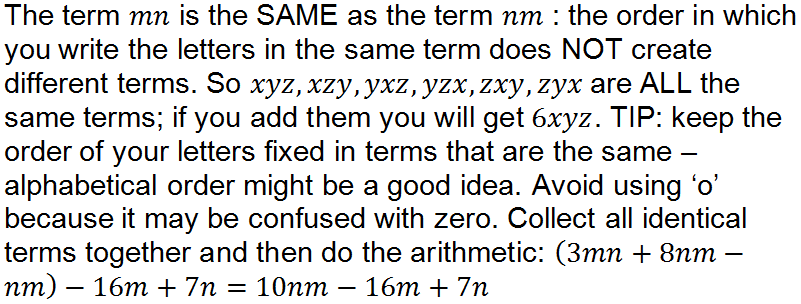Question 3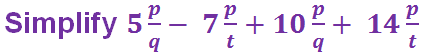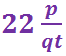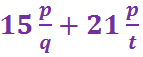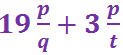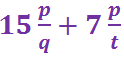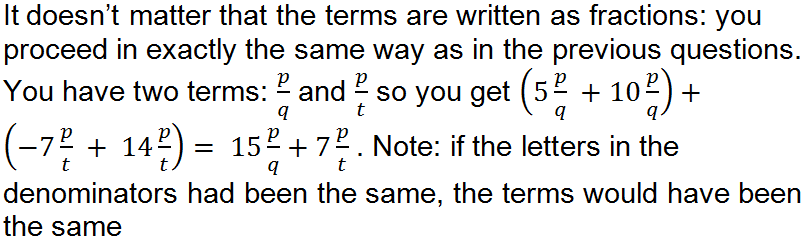Question 4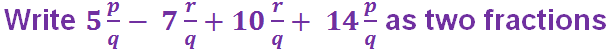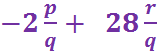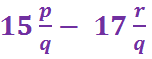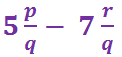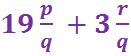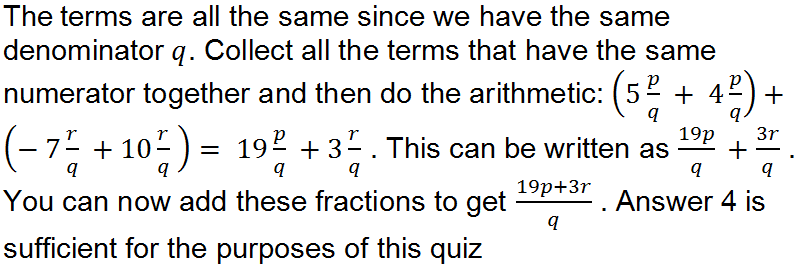Question 5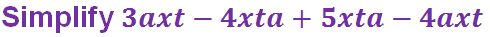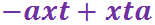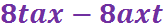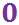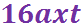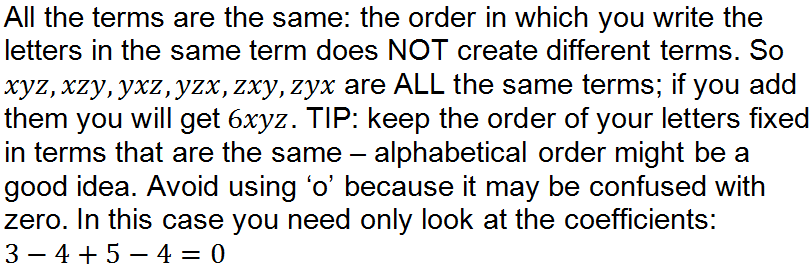Question 6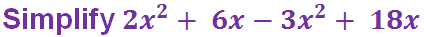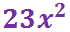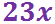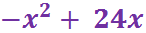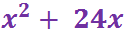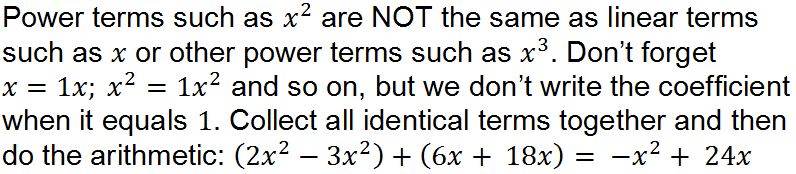Question 7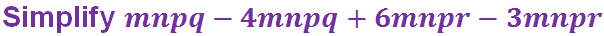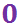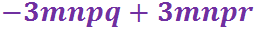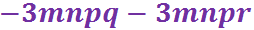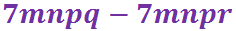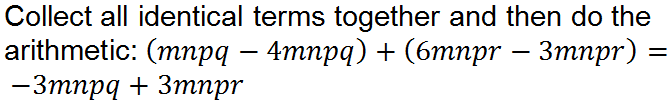Question 8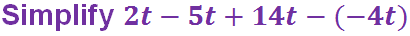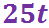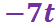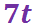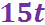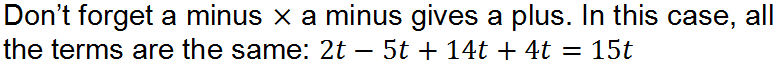Question 9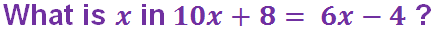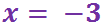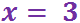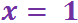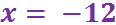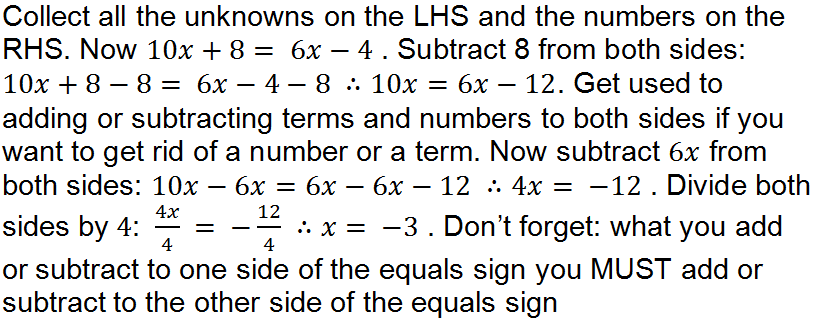Question 10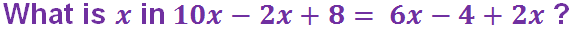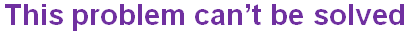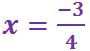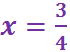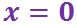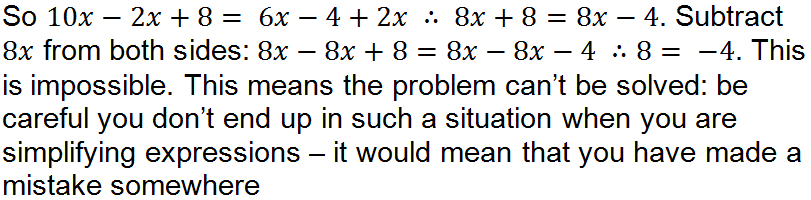Author:  Frank Evans